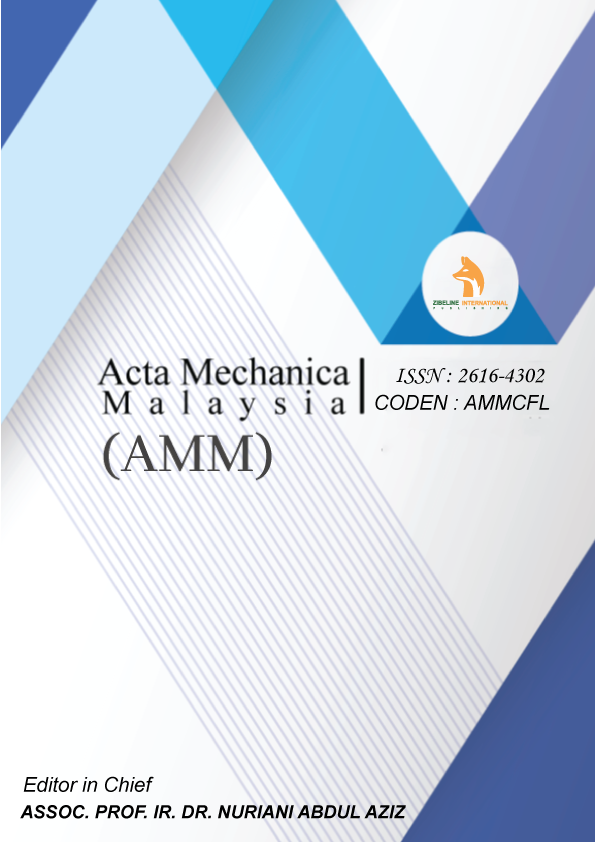##### THE ROLE OF CONCAVE WALLS OF INNER CYLINDER ON NATURAL CONVECTION IN ANNULAR SPACE

ABSTRACT

The objective of the present research is to provide correct results of the roles of physical and geometrical parameters on the natural convection in an annular space of square cylinders. The physical parameters of the fluid are considered as follow: Prandtl number (0.71, 7.01, 50 and 100) and Rayleigh number (10 power 3, and 10 power 4). However, the studied geometrical modification is based on converting the walls of inner square cylinder from the straight form to the concave form. The work is well done numerically. The predicted results are mainly shown as representative contours of streamlines and isotherms. It was understood that the concave walls of inner cylinder reduces the heat transfer rate that can be useful to use this form in insulating applications instead of straight walls.

KEYWORDS

Square annulus, concave walls, square cylinders, heat transfer, and natural convection